#### A car with tire skidding

This section concludes by introducing a car model that considers it as a skidding rigid body in the plane. This model was suggested by Jim Bernard. The C-space is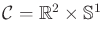, in which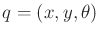. Suppose that as the car moves at high speeds, the tires are able to skid laterally in a direction perpendicular to the main axis of the car (i.e., parallel to the rear axle). Let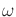denote the angular velocity of the car. Let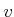denote the lateral skidding velocity, which is another state variable. This results in a five-dimensional state space in which each state is a vector of the form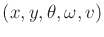.

The position of the rear axle center can be expressed as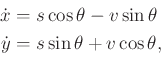(13.110)

which yields two components of the state transition equation. Let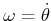denote the angular velocity, which yields one more component of the state transition equation. This leaves only two equations, which are derived from 2D rigid body mechanics (which will be covered in Section 13.3.3). The state transition is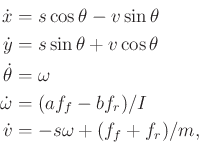(13.111)

in which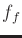and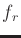are the front and rear tire forces,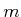is the mass,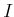is the moment of inertia, and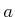and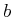are the distances from the center of mass to the front and rear axles, respectively. The first force is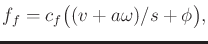(13.112)

in which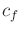is the front cornering stiffness, and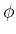is the steering angle. The second force is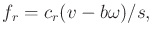(13.113)

in which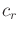is the rear cornering stiffness. The steering angle can be designated as an action variable: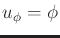. An integrator can be placed in front of the speed to allow accelerations. This increases the state space dimension by one.

Reasonable values for the parameters for an automotive application are: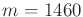kg,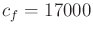,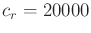,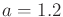m,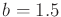m,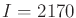kg/m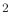, and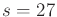m/sec. This state transition equation involves a linear tire skidding model, which is a poor approximation in many applications. Nonlinear tire models provide better approximations to the actual behavior of cars . For a thorough introduction to the dynamics of cars, see .

Steven M LaValle 2020-08-14